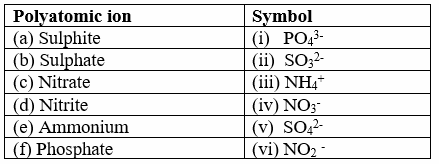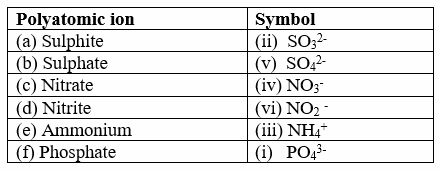# Atoms and Molecules Worksheet

Given below are the Class 9 Science CBSE worksheet for Atoms and Molecules
(a) Short questions
(b) True and False problems
(c) Fill in the blank's
(d) Very Short questions
Hope you like them and do not forget to like , social share and comment at the end of the page.

## Fill In The Blanks

1. During a chemical reaction, the sum of the _________ of the reactants and products remain unchanged.
2. In a pure chemical compound, elements are always present in a ________ proportion by mass.
3. Clusters of atoms that act as an ion are called __________ ions.
4. In ionic compounds, the charge on each ion is used to determine the ________of tye compound.
5. The Avogadro constant _________ is defined as the umber of atoms in exactly ________ of crbon-12
6. Mass of 1 mole of a substance is called its __________.
7. The abbreviation used for length names of elements are termed as their _______.
8. A chemical formula is also known as a ________.
9. Those ions which are formed from single atoms are called _________.
10. Ionic compounds are formed by the combination between ________ an ______.
11. The valency of an ion is _________ to the charge on the ion.
12. Mole is a link between the __________ and __________.
13. The SI unit of amount of a substance is __________.

1. Masses
2. Definite
3. Polyatomic
4. Chemical formula
5. 86.022 x 1023, 12g
6. Molar mass
7. Symbol
8. Molecular formula
9. Simple ins
10. Metal and non- metals
11. Equal
12. Mass of atoms & number of atoms
13. Mole

## True / False

1. Formula mass of $Na_2O$ is 62 amu.
2. Those particles which have more or less electrons than the normal atoms are called ions.
3. Formula for sulphur dioxide is $SO_3$.
4. Molar mass of ethyne ($C_2H_2$) is 26 g/mol.
5. 22 gm of $CO_2$ consists of 1 mole.
6. Number of molecules in 32 gram of oxygen is $6.02 \times 10^{23}$.
7. Water is an atom.
8. Formula for sulphur dioxide is $SO_2$.
9. Clusters of atoms that act as an ion is called polyatomic ion.
10. Mass of 1 mole of a substance is called its formula mass.
11. In a pure chemical compound, elements are always present in a definite proportion by mass.

1. True
2. True
3. False
4. True
5. False
6. True
7. False
8. True
9. True
10. False
11. True

1. Define term mole.

The mole is the amount of substance that contains the same number of particles (atoms/ ions/ molecules/ formula units etc.) as there are atoms in exactly 12 g of carbon-12.

The Avogadro constant $6.022 \times 10^{23}$ is defined as the number of atoms in exactly 12 g of carbon-12.

3.What is gram atomic weight?

Gram atomic weight is the mass in gram of 1 mole of atoms in a mono-atomic chemical element

4. What is the difference between $CO_2$ and $2CO_2$?

$CO_2$ means 1 molecule of carbon dioxide $2CO_2$ means 2 molecules of carbon dioxide

5. Name the following compounds $PC_3$ and $SO_2$.

Phosphate Carbon and Sulphur dioxide

6. In the smallest whole-number ratio must N and O atoms combine to make dinitrogen to tetroxide $N_2O_4$? What is the mole ratio of the elements in this compound?

1:2

7.How many moles of sodium atoms correspond to $1.56 \times 10^{21}$ atoms of sodium?

Sodium atoms = $1.56 \times 10^{21}$
Avogadro number = $6.022 \times 10^{23}$
$Moles = \frac {1.56 \times 10^{21}} { 6.022 \times 10^{23}}$
$Moles = 0.26 \times 10^{-2}$

8. What is the relationship between the formula weight of a substance and its molar mass?

The molar mass of any substance is its atomic mass, molecular mass, or formula mass in grams per mole.

9. How many grams of silver are in 0.263 mol of g?

1 Mole of Silver is 107.86 gm
0.263 mol of silver will be = 107.86 * 0.263=28.367 gm

10.How many atoms are $1.00 \times 10^{-9}$ g of lead?

1 mol of Pb = 207.2g
or 207.2 g has $6.022 \times 10^{23}$ atoms
$1.00 \times 10^{-9}$ will have = $\frac {6.022 \times 10^{23}}{207.2 g} \times {1.00 \times 10^{-9}}=2.91 \times 10^{12}$ atoms.

11.How many grams of iron are needed to combine with 25.6g of O to make $Fe_2O_3$?

Molecular weight of Fe = 55.85
Molecular weight of O = 16.00
Molecular weight of $Fe_2O_3 = (55.85) \times 2 + (16) \times 3 = 111.7+48 = 149.70$
For 48 gm O, Fe required is 111.7 gm
So for 25.6 gm O, Fe required is 59.57 gm

12.What is the mass of 4 moles of aluminium atoms? ( Atomic mass of AI = 27u)

Mass of 1 mole of aluminium atoms = 27 g. Mass of 4 moles of aluminium atoms = (27 x 4) = 108 g.

13.Calculate the mass of $6.022 \times 10^{22}$ atoms of He.

$6.022 \times 10^{23}$ atom of Helium weight 4g
Therefore $6.022 \times 10^{22}$ atoms of Helium will weighs

$= \frac {4 \times 6.022 \times 10^{22}}{6.022 \times 10^{23}} =0.4g$

14.Calculate the number of moles in $3.011 \times 10^{22}$ molecules of carbon dioxide.

$6.022 \times 10^{23}$ is 1 mole
$3.011 \times 10^{22}$ will be .05 mole

15.A sample of 45.8g of $H_2SO_4$ contains how many moles of $H_2SO_4$?

molar mass of $H_2SO_4$ = 98.078 g/mol
No of moles = $\frac {45.8}{98.078}=0.467$ moles

16.What s the mass in grams of 5 moles of Fe?

Molecular mass of Fe= 55.845 g/mol
1 mole of Fe weighs= 55.845g
5 moles of Fe weigh=$55.845 \times 5 =279.225$ g

17.How many moles of NaCI are present in 20 gm of the substance?

Molar mass of $NaCl$ = 58.4 g/mol
Number of moles in 20.0 g NaCl is = $\frac {20}{58.4}= .342$ mol

18.How many grams of O is present in 50 gm of $CaCO_3$?

Molecular mass of $CaCO_3$ = $40 + 12 + 16 \times 3=100$
No of moles in 50 gram = $\frac {50 }{ 100} =0.5$
1 $CaCO_3$ molecule has three oxygen atoms so one mole $CaCO_3$ atom has 3 mole oxygen atom or 48 gm of oxygen
So .5 moles will have = .5 *48 =24 grams of oxygen

19.How many grams of $CO_2$ are present in 0.1 mole $CO_2$?

molecular mass of $CO_2$ = 44
Mass = number of moles * molecular mass
= 0.1 * 44= 4.4 g

20.Describe the difference between the mass of a mole of oxygen atoms (O) and the mass of a mole of oxygen molecules ($O_2$).

Mass of mole of Oxygen atom , i.e one O is present = O = 16 grams
Mass of mole of $O_2$ ,Oxygen gas = $O_2$= 16 + 16 = 32 grams
So a mole of oxygen atoms has only half the mass of a mole of oxygen molecules

21.What do you understand by the term Formula unit?

A formula unit�in chemistry is the empirical formula of any ionic or covalent network solid compound used as an independent entity for stoichiometric calculations. It is the lowest whole number ratio of ions represented in an ionic compound.

Question 1.
Write the name of the compound (NH4)2SO4 and mention the ions present in it?
Question 2.
Why do some atoms react with other atoms to form compounds where as some do not?
Question 3.
Define mole? Calculate the number of moles in 22g of carbon dioxide?
Question 4.
State three rules for writing the chemical formulae.
Question 5.
If one mole of carbon atoms weighs 12 g, what is the mass of 1 atom of carbon?
Question 6.
Derive the formula of the compound formed between Na+ and SO4-2
Question 7.
Write the chemical formulae for the compounds formed by the calcium ion with phosphate, sulphate and hydrogen carbonate ions?
Question 8.
Write the ratio by mass of Hydrogen and oxygen in one molecule of water?
Question 9.
State the number of hydrogen atoms in 1 g of hydrogen?
Question 10.
The relative atomic mass of oxygen atom is 16. Explain its meaning?
Question 11.
Calculate the number of molecules of SO2 present in 44 g of it?
Question 12.
If one molecule of oxygen atoms weighs 16 g, find the mass of one atom of oxygen?
Question 13
The atomic mass of calcium is 40 u. What will be the number of calcium atoms in 0.4 of calcium?
Question 14.
Molar mass of nitrogen is 14 u. What will be the mass of one atom of nitrogen?
Question 15.
Calculate the number of aluminium ions present in 0.051 g of aluminium oxide?
Question 16.
Calculate the number of molecules of sulphur (S-8) present in 8g of solid sulphur?
Question 17.
State any four postulates of Dalton’s atomic theory of matter. Which of his postulates does not hold correct at present?

## Match the column

Match the Column I to Column II## Summary

This Class 9 Science worksheet for Atoms and Molecules with answers is prepared keeping in mind the latest syllabus of CBSE . This has been designed in a way to improve the academic performance of the students. If you find mistakes , please do provide the feedback on the mail.